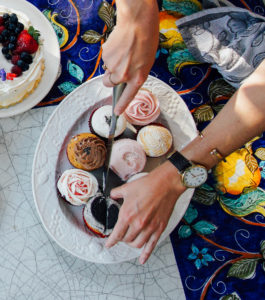## Putting It Together: Whole Numbers, Fractions, Decimals, Percents, and Problem Solving

Let’s revisit the math you’ll need to make decisions for Samuel’s graduation party.

You determine that you will need five boxes of cupcake mix to make enough for the crowd. Sweet Sprinkles cake mix boxes are $2$ for $$5.50$, or $1$ for$$3.75$. Chocolover cake mix boxes are $3$ for $$6.99$, or $1$ for$$2.55$. What is the most economical way to purchase what you need?All Sweet Sprinkles: $(2\cdot{5.50})+3.75=\14.75$

All Chocolover: $(2\cdot{2.55})+6.99=\12.09$

Combination of brands: $5.50+6.99=\12.49$

You will need to triple your frosting recipe, which calls for 3/4 cup of cream cheese, 1/3 tsp of vanilla, and 2.5 cups of powdered sugar. How much of each ingredient will you need?

Cream cheese: $3\cdot(\frac{3}{4})=(\frac{3}{1})(\frac{3}{4})=\frac{9}{4}$ or $2\frac{1}{4}$ cups

Vanilla: $3\cdot(\frac{1}{3})=(\frac{3}{1})(\frac{1}{3})=\frac{3}{3}$ or $1$ teaspoon

Powdered sugar: $2.5=\frac{5}{2}$; $3\cdot(\frac{5}{2})=(\frac{3}{1})(\frac{5}{2})=\frac{15}{2}$ or $7\frac{1}{2}$ cups

You have invited 96 people to the party and guess that only about 76% will be able to show up. First, figure out how many people you think will attend the party (round to the ones place — whole person). According to what you’ve read online, people consume about 5 drinks per person at a party. If drinks come in 12-packs, how many packs will you need to buy to cover the estimated drink needs?

Attendees: $96\cdot(.76)=72.96$ rounded to $73$ whole people

Drinks needed: $73\cdot5=365$ drinks

12-packs to buy: $365/12\approx{30.41667}$ rounded up to $31$ packs to cover the estimated drink needs

You have six friends who are willing to split the cost of food, drinks, and decorations as long as you cover the dishes and utensils. The total cost for everything was $\480.39$ and the plates, napkins, spoons, etc. added up to $\125.61$. How much will each of the friends need to pay you for their share?

Cost of food, drinks, decorations: $\480.39-\125.61=\354.78$

Split cost: $\354.78/6=\59.13$

As you can see, the math we reviewed in the module is extremely useful in a making calculations and decisions for a scenario like a big graduation party!

## Contribute!

Did you have an idea for improving this content? We’d love your input.